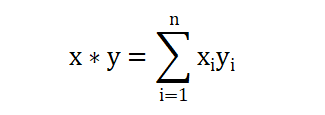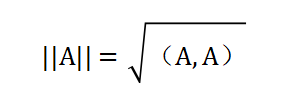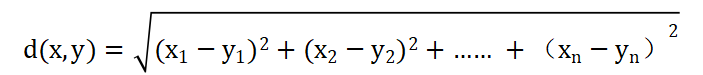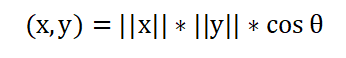1594字，阅读需时6分钟（1）(x,y) = (y,x)

（2）(x,y+z) =(x,y)+(x,z)

（3）c(x,y) = (cx,y)

（4）若x!=0，则(x,y)>0

import numpy as np

# 定义三维空间的x,y,z向量
x = np.array([1,-1,3])
y = np.array([2,-2,1])
z = np.array([-2,3,1])

#验证交换性
dot1 = np.dot(x,y)
dot2 = np.dot(y,x)
print("验证交换性")
print("(x,y)=%.2f：(y,x)=%.2f" % (dot1,dot2))
#验证分配性
dot1 = np.dot(x,y+z)
dot2 = np.dot(x,y) + np.dot(x,z)
dot3 = np.dot(x,y) + np.dot(y,z)
print("验证分配性")
print("(x,y+z)=%.2f：(x,y)+(x,z)=%.2f" % (dot1,dot2))
#验证结合性
# 定义任意实数c
c = 0.2
dot1 = c*np.dot(x,y)
dot2 = np.dot(c*x,y)
dot3 = np.dot(x,c*y)
print("验证结合性")
print("c(x,y)=%.2f：(cx,y)=%.2f: (x,cy)=%.2f" % (dot1,dot2,dot3))